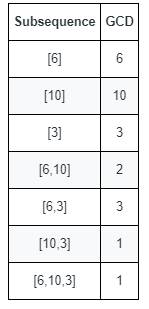Formatted question description: https://leetcode.ca/all/1819.html

# 1819. Number of Different Subsequences GCDs

Hard

## Description

You are given an array nums that consists of positive integers.

The GCD of a sequence of numbers is defined as the greatest integer that divides all the numbers in the sequence evenly.

• For example, the GCD of the sequence [4,6,16] is 2.

A subsequence of an array is a sequence that can be formed by removing some elements (possibly none) of the array.

• For example, [2,5,10] is a subsequence of [1,2,1,2,4,1,5,10].

Return the number of different GCDs among all non-empty subsequences of nums.

Example 1:Input: nums = [6,10,3]

Output: 5

Explanation: The figure shows all the non-empty subsequences and their GCDs.

The different GCDs are 6, 10, 3, 2, and 1.

Example 2:

Input: nums = [5,15,40,5,6]

Output: 7

Constraints:

• 1 <= nums.length <= 10^5
• 1 <= nums[i] <= 2 * 10^5

## Solution

For each num in nums, obtain all the factors of num. Count the number of each factor from 1 to max(nums). For each i such that 1 <= i <= max(nums), let counts[i] be the number of factor i that occurs in nums. If i is in nums, or counts[i] > 0 and there does not exist any j such that j > i and j % i == 0, then i is the GCD of a subsequence of nums. Finally, return the number of different subsequences GCDs.

class Solution {
public int countDifferentSubsequenceGCDs(int[] nums) {
Set<Integer> set = new HashSet<Integer>();
int max = 0;
for (int num : nums) {
max = Math.max(max, num);
}
int[] counts = new int[max + 1];
for (int num : set) {
for (int i = 1; i * i <= num; i++) {
if (num % i == 0) {
counts[i]++;
if (i * i != num)
counts[num / i]++;
}
}
}
int count = 0;
for (int i = 1; i <= max; i++) {
if (set.contains(i))
count++;
else if (counts[i] > 0) {
boolean flag = true;
for (int j = i * 2; j <= max; j += i) {
if (counts[j] == counts[i]) {
flag = false;
break;
}
}
if (flag)
count++;
}
}
return count;
}
}